Nyquist's theorem, also known as the sampling theorem, states that a periodic signal must be sampled at more than twice the highest frequency component of the signal.

In imaging, we recommend sampling at least 2.3X the highest frequency. What does this mean practically?

Nyquist conditions can be applied to many imaging parameters, X, Y, Z, and time.

Let's look at this first example where we collect an image over time. In other words, for a cell with diameter of 10 um that moves at 5 um/ minute, calculation: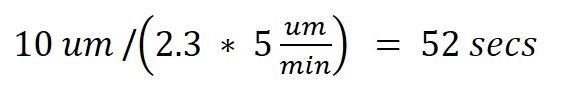Therefore, we need to sample the data with a time resolution of a minimum of 52 seconds to be able to capture the movement correctly.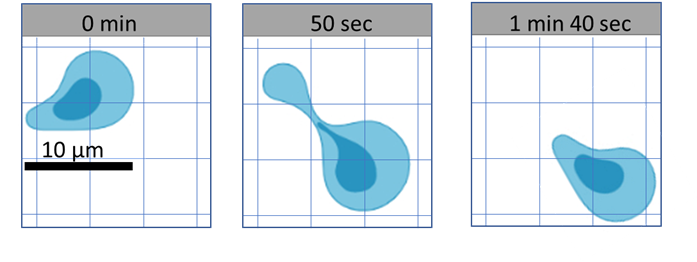Here, we are able to visualize the cell moving from the top left corner, to the bottom right corner and reliably determine it is the same cell.

However, if we collect an image every 2 minutes, we cannot determine if this is the same cell, or if these are 2 different cells.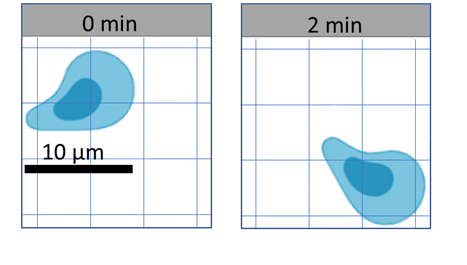However, if instead of tracking the entire cell, we are interested in tracking the nucleus which is 5 um in diameter, then we would need to collect another image every 26 seconds.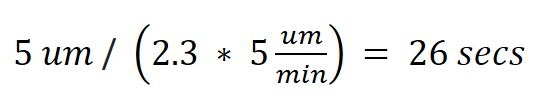Likewise, if the width of your cell is 10 um, you need XY resolution to be less than 5um to be able to resolve 2 cells next to each other.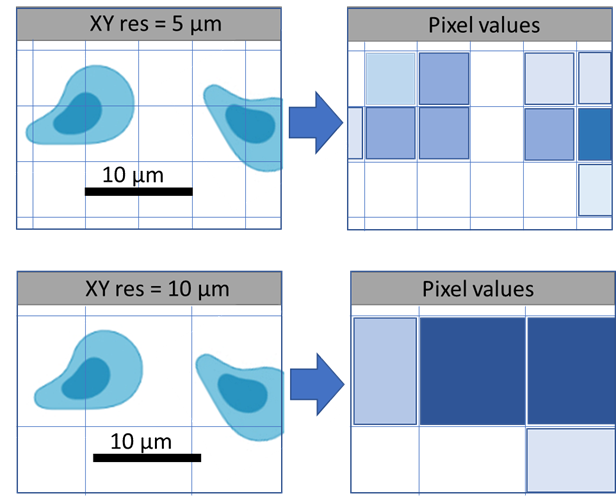Resolution is the ability of an objective to definitively resolve an object, or group of objects, as a single entity(s). Magnification plays no part in the ability of an objective to resolve an object of a given size. While magnification will make an image bigger, it will not necessarily make it clearer. Resolution is governed solely by the Numerical Aperture (NA) of the objective, the wavelength of light and for axial resolution, the refractive index of the sample. Magnification will affect how much light the objective can collect. Higher magnification objectives collect less light, so where possible use the highest NA, lowest magnification objective available.

It is important when discriminating small structures that the Nyquist Sampling Criteria is satisfied. Nyquist Sampling dictates that to optimally represent an analogue signal in digital space, the analogue signal needs to be sampled at least 2.3 times. In microscopy terms this means that the pixel size of an image needs to be at least 2.3 times smaller than the object that is being resolved.

If you are attempting to capture the highest resolution image possible with a given microscope configuration (objective, camera/scanner, excitation and emission wavelength etc) then you must ensure that the pixel size is at least 2.3 times smaller than the calculated resolution of the objective.

On a wide field microscope equipped with a digital camera the pixel size is usually fixed for each objective and cannot be adjusted. The software used to take an image with the camera will show what the pixel size is, usually it is small enough to achieve the theoretical maximum resolution of the objective. It is important to make sure you are aware of the pixel size for widefield imaging.

On a confocal microscope the pixel size can be adjusted. This is achieved by scanning at a higher image resolution (e.g. 1024x1024 instead of 512x512). If there are more pixels in the image each one has to represent a smaller area. Adjusting the zoom will result in the pixel being smaller as well, at the cost of a smaller sample area imaged.

It is also important to apply Nyquist sampling rates to Z-stacks as well as timelapse imaging. For z-stacks, it is important that the user sets the z-step size to 2.3 times smaller than the Z-resolution of the objective. The Zeiss confocal microscopes have inbuilt calculators, use the Optimal settings when setting XY resolution and Z stacks.  Nikon decon also has an Optimal setting for Z stacks.

Microscope vendors tend to use 2x oversampling instead of the recommended 2.3x to 3x oversampling required for image deconvolution, so you may want to reduce the step size a bit smaller than recommended, or refer to the following resources for the optimal step size.

Refer to the Nyquist calculator to determine the correct XYZ sampling rates (pixel sizes) for your images.

Android app: https://svi.nl/NyquistApp

Ref: Handbook of biological confocal microscopy / edited by James B. Pawley